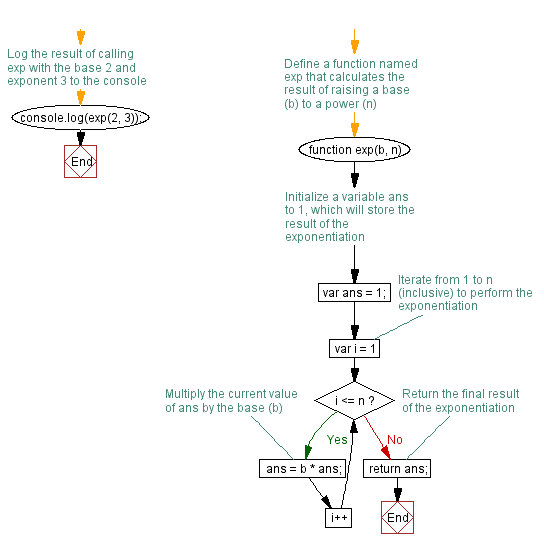# JavaScript: Compute the value of bn where n is the exponent and b is the bases

## JavaScript Function: Exercise-15 with Solution

Write a JavaScript function to compute the value of bn where n is the exponent and b is the bases. Accept b and n from the user and display the result.

Sample Solution: -

HTML Code:

``````<!DOCTYPE html>
<html>
<meta charset=utf-8 />
<title>Compute the value of bn where n is the exponent and b is the bases</title>
<body>
</body>
</html>
```
```

JavaScript Code:

``````function exp(b,n)
{
var ans = 1;
for (var i =1; i <= n; i++)
{
ans = b * ans;
}
return ans;
}
console.log(exp(2, 3));
```
```

Sample Output:

```8
```

Flowchart:Live Demo:

See the Pen JavaScript - Compute the value of bn where n is the exponent and b is the bases-function-ex- 15 by w3resource (@w3resource) on CodePen.

Improve this sample solution and post your code through Disqus

What is the difficulty level of this exercise?

﻿

## JavaScript: Tips of the Day

Creates a function that invokes fn with a given context, optionally adding any additional supplied parameters to the beginning of the arguments

Example:

```const bind = (fn, context, ...boundArgs) => (...args) => fn.apply(context, [...boundArgs, ...args]);
function tips_greet(greeting, punctuation) {
return greeting + ' ' + this.user + punctuation;
}
const w3r = { user: 'Owen' };
const w3rBound = bind(tips_greet, w3r);
console.log(w3rBound('hi', '!'));
```

Output:

```hi Owen!
```